# Sesja plakatowa

Eugeniusz Barcz (UWM w Olsztynie): Local versions of Banach principle and new methods of applications of contractive maps

In the paper „Local versions of Banach principle and new methods of applications of contracive maps” in a shape of a poster we demonstrate generalizations of local versions of the Banach principle for nonlinear contractions adding new methods of applications of contractive maps.

Generalizations of local versions of Banach principle related to the so called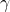-contractions are shown being the main results of this paper. The famous Cauchy problem is considered here for differential equation in Banach space. In the proof of this theorem we use the new method based on some local version of Banach principle (Theorem 2.5)

In this paper it also proved the inverse function theorem both in Banach spaces. The proofs presented here, based on the local versions of Banach principle, are essentially simpler.

[PDF]

Dorota Bors (Uniwersytet Łódzki): Stability of Dirichlet problems involving fractional Laplacian

We consider some Dirichlet problems for the differential equations with fractional Laplacian. Both existence and stability results are proved by the use of the varational methods.

[PDF]

Antoni Leon Dawidowicz (Uniwersytet Jagielloński): Delayed differential equation with non-constant delay in biology

Differential equations with delayed argument have numerous applications in biology, like e.g. in immunology, whether of epidemiology. These equation has the formwhere the set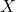of values of function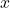may be olso multidimensional. We shall present the applications of more general equations i. e. equations of the formwhere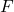is defined on function space andis defined by the formulaThe examples of application of such equations will be presented. To such equations the classical method of steps cannot be used.

Co-authors: Anna Poskrobko (Uniwersytet w Białymstoku), Jerzy Leszek Zalasiński (Expert FAO)

[PDF]

Danuta Jaruszewska-Walczak (Uniwersytet Gdański): Method of lines for Sobolev type equations

We consider the first initial boundary value problem for the following nonlinear Sobolev type equation with a rapid growing nonlinearityHereis a bounded domain with smooth boundary and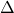is the Laplace operator with respect to the spatial variable. The unique solvability in the classical sense for this problem is proved by M. O. Korpusov and A. G. Sveshnikov. Estimates for the time of the blow-up are given.

We are interested in numerical solving such problem. Stability of the method of lines is investigated.

[PDF]

Adrian Karpowicz (Uniwersytet Gdański): On the Cauchy problem for hyperbolic functional-differential equations

We consider the Cauchy problem for a nonlocal wave equation in one dimension. We study the existence of solutions by means of bicharacteristics. The existence and uniqueness is obtained intopology. The existence theorem is proved in a subset generated by certain continuity conditions for the derivatives.

[PDF]

Katarzyna D. Lewandowska (GUMed): Applications of differential equations with fractional time derivative in describing subdiffusion processes

We show the applications of hyperbolic and parabolic subdiffusive equation with time fractional derivative to describe the transport process in membrane system and to study the subdiffusive impedance in electrochemical system. Based on solutions of the equations we find characteristic power functions which can be used to extract the subdiffusive parameters of the system from experimental data. To illustrate our considerations we find the values of subdiffusion parameters for a few media.

[PDF]

Magdalena Nockowska-Rosiak (Politechnika Łódzka): Bounded and stable solutions for nonlinear second order neutral difference equation

Using the techniques connected with the measure of noncompactness we investigate the neutral difference equation of the following formwhere,,,is continuous and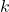is a given positive integer,is a ratio of positive integers with odd denominator, andis ratio of odd positive integers;. Sufficient conditions for the existence of a bounded or stable of a special type solution are presented.

Co-authors: Marek Galewski, Robert Jankowski (Politechnika Łódzka)
Robert Jankowski, Ewa Schmeidel (Uniwersytet w Białymstoku)

[PDF]

Krzysztof A. Topolski (Uniwersytet Gdański): Generalized upper and lower solutions for discontinuous ordinary differential equations

First order discontinuous ordinary differential equations are considered. By considering noncontinuous sub and supersolutions (upper and lower absolutely continuous functions) we obtain more general results than in standard theory. We present theorems on the existence of extremal solutions for a large class of boundary value problems. We assume neither continuity nor monotonicity of boundary functions.

[PDF]

Rafał D. Urniaż (Uniwersytet Medyczny w Lublinie): The APD-RMSD descriptor for molecules' and ligands' conformation

[PDF]

==================

Damian Wiśniewski (UWM w Olsztynie): Integro - differential inequalities related to the least positive eigenvalue of some eigenvalue problems

We consider the eigenvalue problem for the Laplace-Beltrami operatoron the unit sphere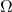:and for– Laplacian Dirichlet problem:We have proved the existence of the smallest positive eigenvalue ofand Friedrichs-Wirtinger-type inequalities corresponding to our problems.
We have also derived some integro-differential inequalities related to the smallest positive eigenvalue ofand.

Co-author: Mariusz Bodzioch

[PDF]

Monika Wrzosek (Uniwersytet Gdański): Method of lines for hyperbolic stochastic functional partial differential equations

We consider the initial value problem for first-order stochastic functional partial differential equation driven by Brownian motionwhereis white noise andis a Hale-type operatorWe apply the method of lines and prove the stability of the numerical scheme. This result is proved with the help of representation, existence and uniqueness, and the estimation of solution lemmas.

Co-author: Maria Ziemlańska

[PDF]

Małgorzata Zdanowicz (Uniwersytet w Białymstoku): Nonoscillatory bounded solutions of k-dimensional system of neutral difference equations

[PDF]

Krzysztof Żyjewski (UWM w Olsztynie): Nonlocal Robin problem for weak quasilinear elliptic equations in a plane domain

Letbe a bounded domain. We assume that the boundaryis a smooth curve everywhere except at the originand near the pointcurvesare lateral sides of an angle with the measureand the vertex atnearthe curveWe shall consider a weak quasilinear elliptic equation with the nonlocal boundary condition connecting the values of the unknown function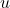on the curveswith its values ofon the()here:

••are diffeomorphisms mapping ofontoWe investigate the behavior of weak solutions of the above problem in a neighborhood of the boundary corner point.

[PDF]